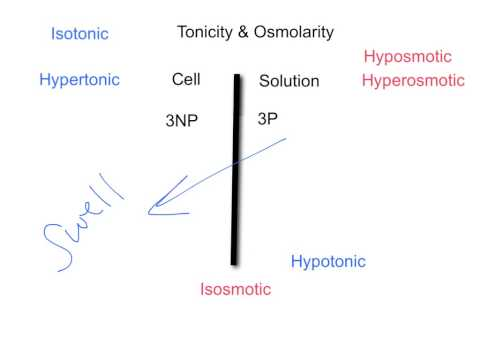Key Difference

The terms Osmolarity and Tonicity are interrelated as one is most oftenly talked when the other is being discussed. Though, beside this closeness, one can easily differentiate it between both the terms. Osmolarity is the concentration of a solution expressed as the total number of solute particles per litre, whereas Tonicity is the measure of the effective atmospheric gradient and the ability of the extracellular solution to make solution move into or out of a cell with the process of Osmosis. Osmolarity takes all of the solute concentrations under consideration, while tonicity only considers the solutes that failed to pass through the semipermeable membrane as they are the only solutes having an influence on osmotic pressure gradient.

### Comparison Chart

 Osmolarity Tonicity Definition Osmolarity is the concentration of a solution expressed as the total number of solute particles per litre. Tonicity is the measure of the effective atmospheric gradient and ability of the extracellular solution to make solution move into or out of a cell with the process of Osmosis. Concentration Osmolarity takes all of the solute concentrations under consideration. Tonicity only considers the solutes that failed to pass through the semipermeable membrane. Units of Measurement osmol/L mol/L

What is Osmolarity?

Osmolarity is the concentration of a solution expressed as the total number of solute particles per litre. It considers all the solutes in the solution whether permeable or non-permeable. It is the measurement of solute concentration per unit of volume, and it is denoted using osmoles present in the solution. In the majority of the cases, the value for osmolarity is similar to the value of molarity. Though they are quite different as osmolarity involves the total amount of solutes in a solution whereas the molarity is about the entire concentration of the compound. The Osmolarity can further be divided into three forms relative the osmotic pressure of the solution. 1) Isosmotic Solution: The Atmospheric pressure of the solution is equal to the surrounding. 2) Hyperosmotic Solution: The Atmospheric pressure of the solution is higher than the surrounding. 3) Hypoosmotic Solution: The Atmospheric pressure of the solution is lower than the surrounding.

What is Tonicity?

Tonicity is the measure of the effective atmospheric gradient and ability of the extracellular solution to make solution move into or out of a cell with the process of Osmosis. Unlike osmolarity, tonicity only considers the solutes that failed to pass through the semipermeable membrane as they are the only solutes having an influence on osmotic pressure gradient. Solutes freely crossing the membrane have no affect on tonicity because they always remain in equal concentrations on both sides of the membranes. Tonicity is often misunderstood when it is called the measure of the osmotic pressure itself. Actually, it is the measure of the osmotic pressure gradient. Generally, it is utilized while describing the response of cells immersed in an external solution.

Osmolarity vs. Tonicity

• Osmolarity is the concentration of a solution expressed as the total number of solute particles per litre, whereas Tonicity is the measure of the effective atmospheric gradient and ability of the extracellular solution to make solution move into or out of a cell with the process of Osmosis.
• Osmolarity takes all of the solute concentrations under consideration, while tonicity only considers the solutes that failed to pass through the semipermeable membrane.
• Osmolarity is expressed as osmol/L, whereas tonicity is denoted using mol/L.